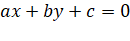Mathematics SSS 2 First Term

Theme: Numbers and Numeration

WEEK 10

Performance Objectives

Students should be able to;

1. Define graph and identify the x-intercept and y-intercept of any linear graph

1. Draw the graph of linear equation ax+by+c=02. Draw the graph of a linear equation by using the gradient method
3. Find the equation of a line using the point quadratic equation.

Straight Line Graph

Content

Definition of Graph

A graph is a picture that represents numerical data. Most of the graphs that you have been taught are straight-line or linear graphs. This topic shows how to use linear graphs to represent various real-life situations.

Note:

1. If the rule.... View More